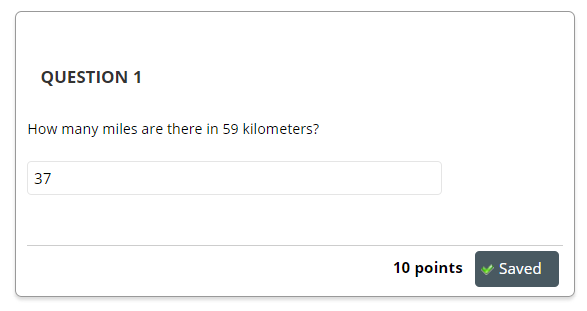# Calculated Formula Questions

A Calculated Formula question contains a formula, the variables of which can be set to change for each user. The variable range is created by specifying a minimum value and a maximum value for each variable. Answer sets are randomly generated using a set of criteria you provide.

 There is a known issue when using exponential numbers within Calculated Formula Questions, see bottom of this page. When creating answer sets, specify to use decimal places rather than significant figures, see more at step 9 below.

1. From your test canvas, choose Calculated Formula from the Create Question menu.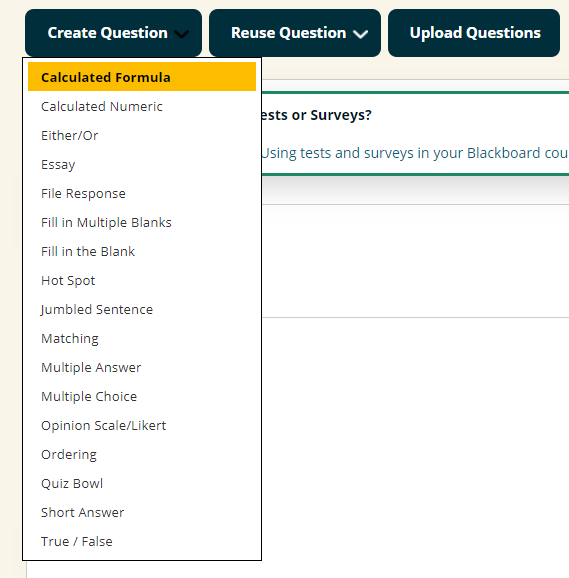2. On each screen and at each stage pay careful attention to the instructions shown.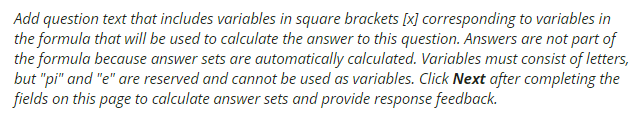3. Adding a question title is optional.  Enter the question text.  Surround any variables with square brackets, for example, [n]. The value for this variable will be populated based on the formula we create in the next part.

Please note that Blackboard currently advise that this question type does not function correctly if the question is created with five or more variables.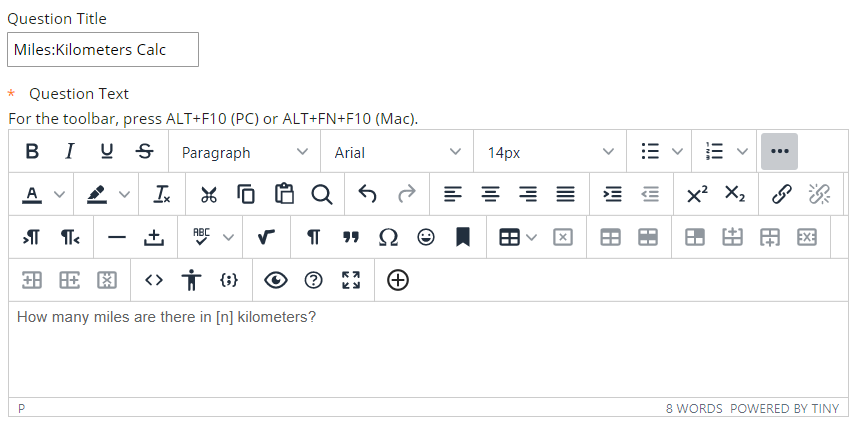4. Enter the formula used to answer the question.  Different operations are selectable using the buttons above the text.  For the variables use the same letters that are enclosed in square brackets in your question text.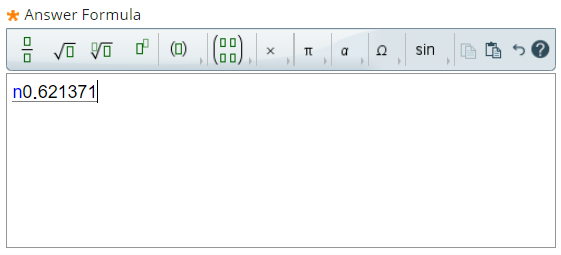5. Choose the options to suit your preferences.

This defines which submitted answers will be marked correct. If the exact value must be entered, enter 0 and select Numeric from the drop-down list. If the answer can vary, enter a value and select Numeric or Percent. Numeric will mark every answer as correct that falls within a range of plus or minus the Answer Range from the exact answer. Percent will mark every answer as correct that falls within a percentage of plus or minus the Answer Range from the exact answer.

Allow Partial Credit
Define partial credit for answers that fall outside the correct Answer Range. Select Yes or No for Allow Partial Credit. Enter a value for the Partial Credit Points Percentage. Now, set the range for partial credit by entering a value and selecting Numeric or Percent for the Partial Credit Range. Answers falling within this range will receive a portion of the total points possible for the question equal to the Partial Credit Points Percentage.

Units Required
Select Yes or No for Units Required. If Yes, correct answers must include the correct unit of measurement, for example, Seconds or Grams. Enter the correct unit of measurement and choose if the unit of measurement is case sensitive. The answer may still receive partial credit if the unit of measurement is not correct. Enter a percentage in Unit Points Percentage. The unit of measurement will account for that percentage of the total credit.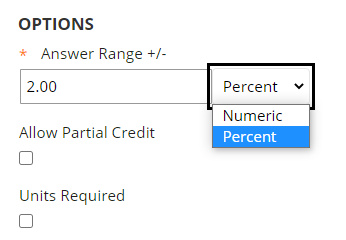6. Select Next to continue.

7. You will see a list of all the variables entered in the question text.  Choose the minimum and maximum values for each variable.  These will be used to build the answer set.

8. Choose how many decimal places should be used for each variable.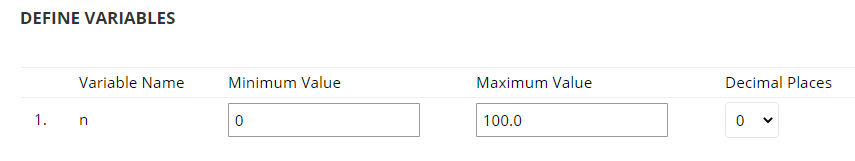9. Choose the number of Decimal Places for Answers. This determines the number of decimal places a student answering the question is expected to use.

You can change this to significant figures but this is not recommended.  If you specify significant figures, then students have to give an answer with the same number of significant figures that you specify, but unless you state this in the question text, they will not know that they need to do so.

10. Set the Number of Answer Sets. This means the number of possible variables that will be calculated at the next stage. The Answer Sets will be randomised so that different Students will be presented with a different set of variables.

Set the Correct Answer Format: the choices are normal or exponential.  Note the known issue at the end of this page.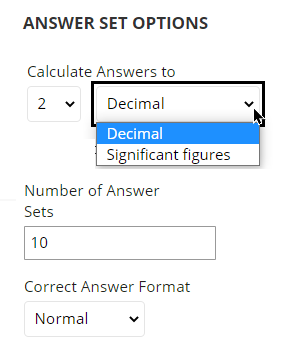11. Select Calculate to calculate the answer set.

12. The number of answer sets you specified previously will be displayed.  You can remove any if you wish.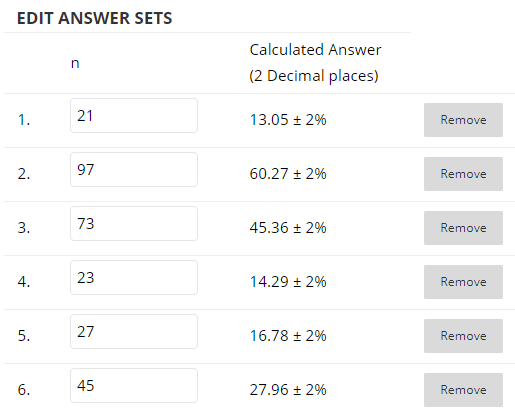13. Enter Feedback for correct and incorrect answers.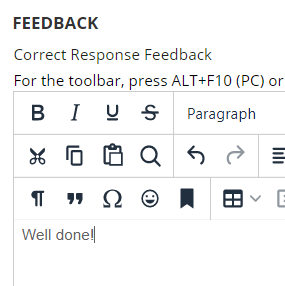14.  If you wish you may set categories and keywords for your question.  This is not necessary for the question to work.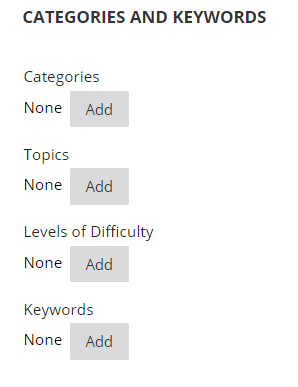15.  Add any “instructor notes” if you wish.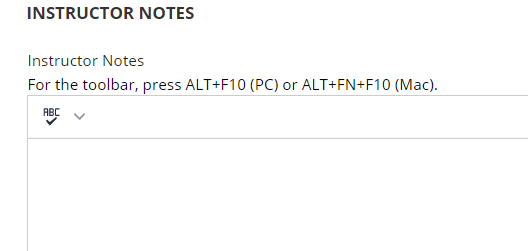16. Select Submit to finish.

17. You will see your final question listed on the test canvas.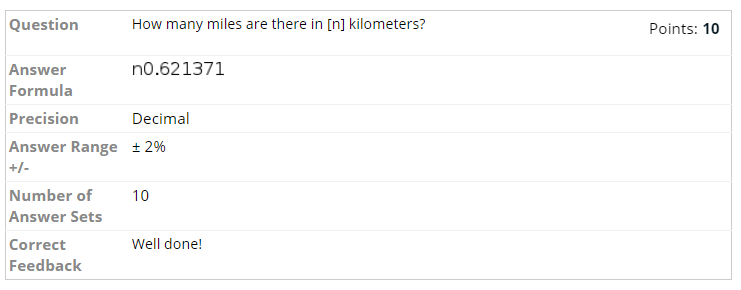17.  This is how the question looks when a student answers it.